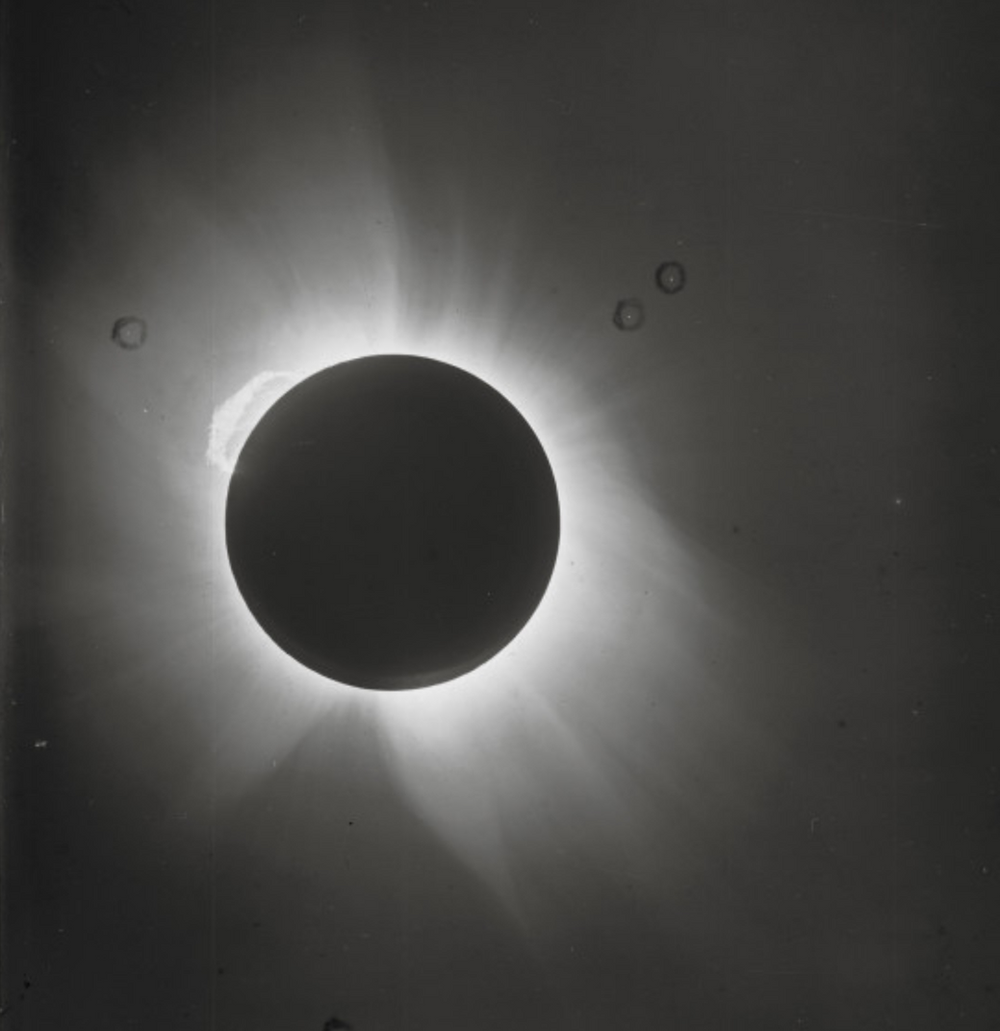Search

# The Eclipse that Made Einstein FamousThis photo made Einstein the national Icon he was, and still is today. But why? What's so special about a solar eclipse? There are many of them that happen all the time.

In the early 1900's, Albert Einstein proposed that Newtons idea of gravity, first proposed in his Principia Mathmatica published in 1687, was incorrect. That would mean that for over 200 years the world didn't quite understand the true meaning of gravity and its role in space. Newton's law states that "every point mass attracts every other point mass by a force acting along the line intersecting the two points. The force is proportional to the product of the two masses, and inversely proportional to the square of the distance between them." In other words:This would mean that gravity is universal and constant. Einstein saw a flaw in the theory, in that, he believed gravity wasn't the attraction between objects, but instead, the influence of objects on space itself, bending space like a cloth. In 1919, he set out to try and prove him wrong. If Einstein were right, then the same mathematics that prove his idea of bending space would also apply to the light of distant stars. Einstein proposed an ingenious and clever way of measuring bending light, by using a total solar eclipse.If light were to bend around the sun, then all you would need to do is observe some bright stars in the location th